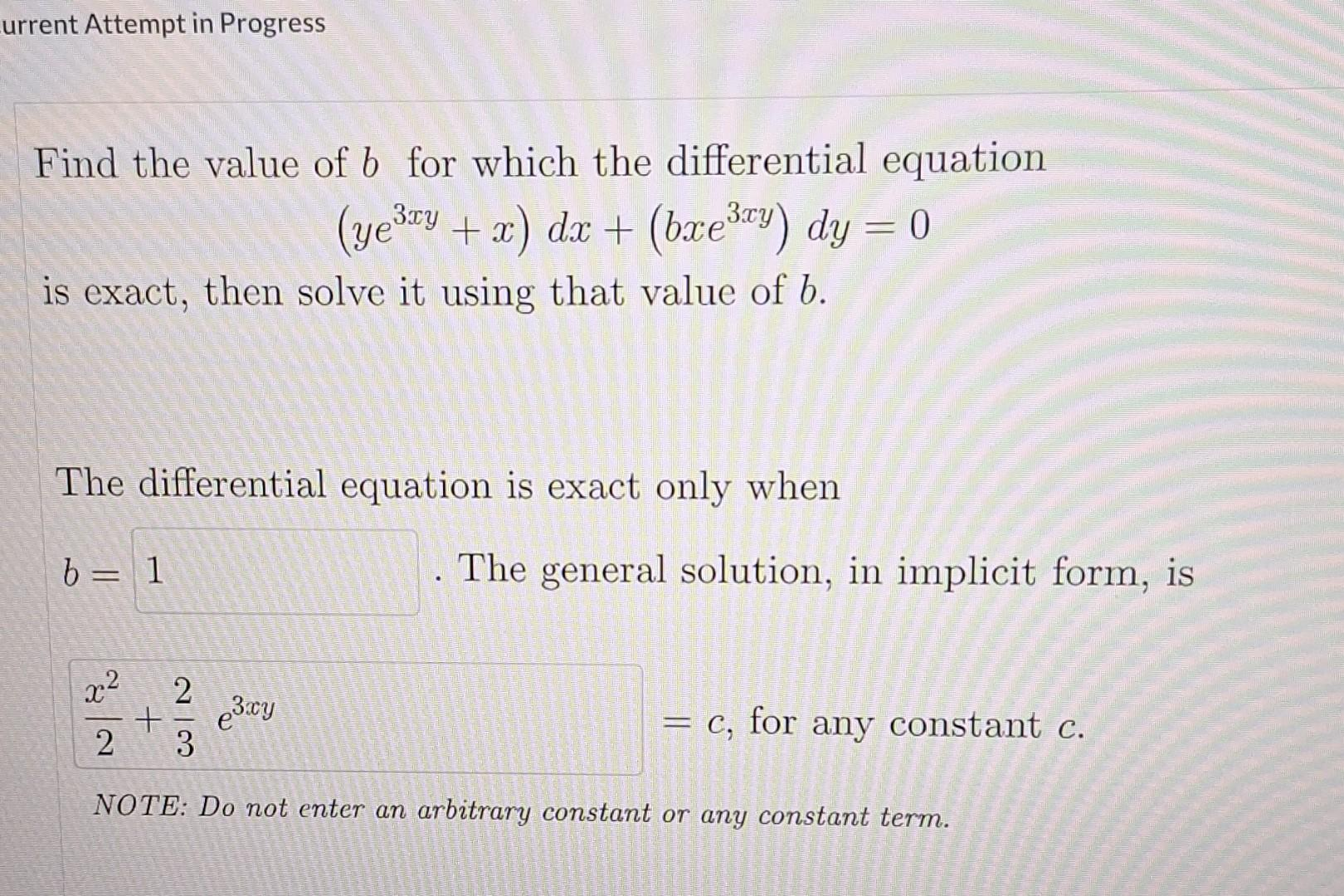Home / Expert Answers / Calculus / find-the-value-of-b-for-which-the-differential-equation-yey-x-dx-bxey-dy-0-3xy-is-pa571

# (Solved): Find the value of b for which the differential equation (yey + x) dx + (bxey) dy = 0 3xy is ...

Find the value of b for which the differential equation
(ye³y + x) dx + (bxe³y) dy = 0
3xy
is exact, then solve it using that value of b.
The differential equation is exact only when
b = 1

2
+
2/3
3xy
The general solution, in implicit form, is
= c, for any constant c.
NOTE: Do not enter an arbitrary constant or any constant term.Find the value of for which the differential equation is exact, then solve it using that value of . The differential equation is exact only when . The general solution, in implicit form, is NOTE: Do not enter an arbitrary constant or any constant term.

We have an Answer from Expert